maths > mensuration-basics

Surface Area of Cube, Cuboid, Cylinder

what you'll learn...

Overview

Surface Area of Some Shapes: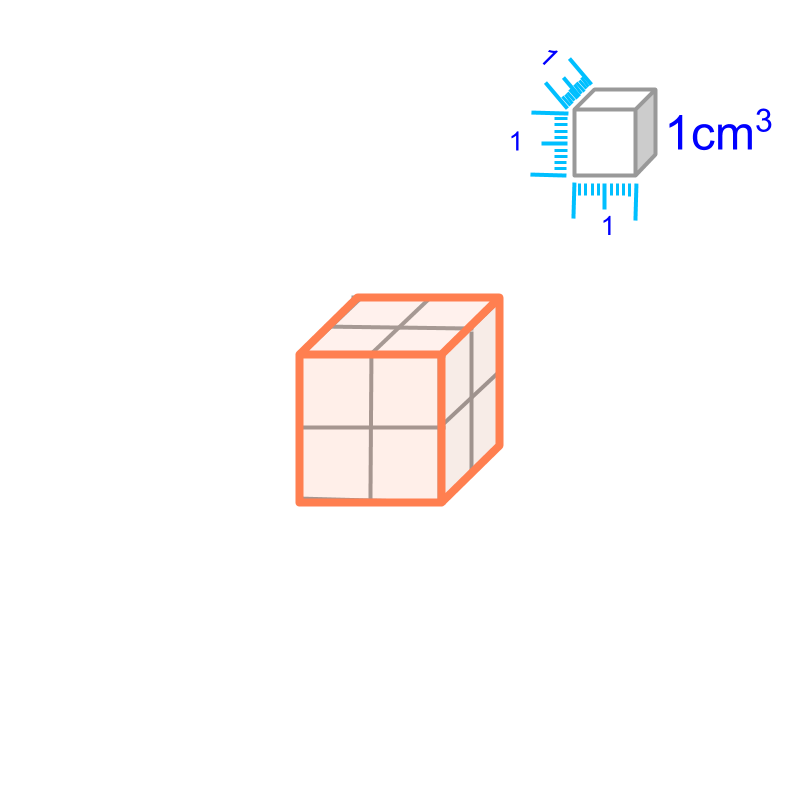Surface Area of cube $=6{q}^{2}$$= 6 {q}^{2}$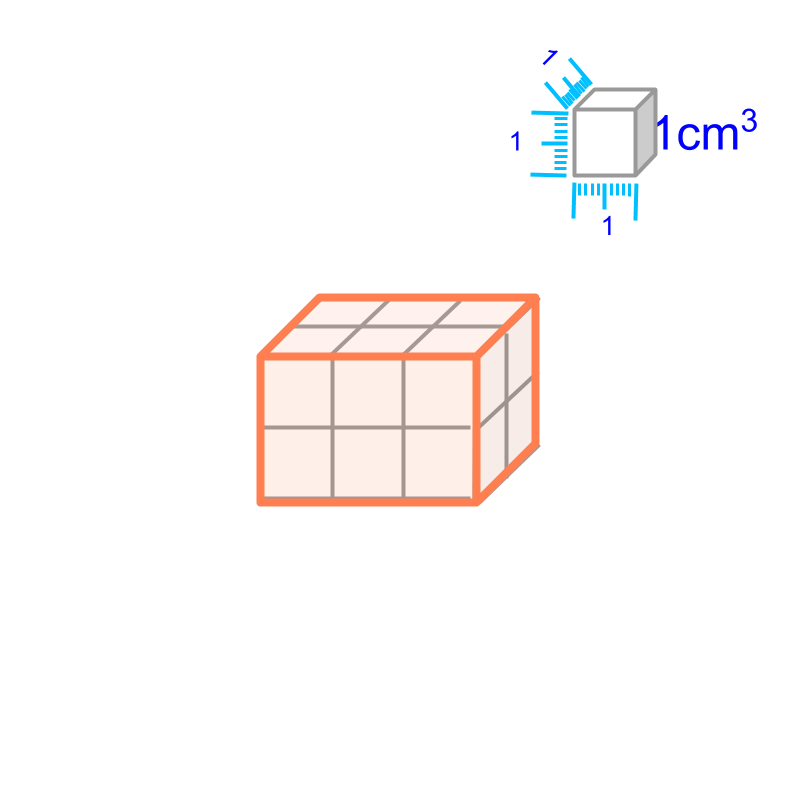Surface Area of cuboid $=2\left(lb+bh+hl\right)$$= 2 \left(l b + b h + h l\right)$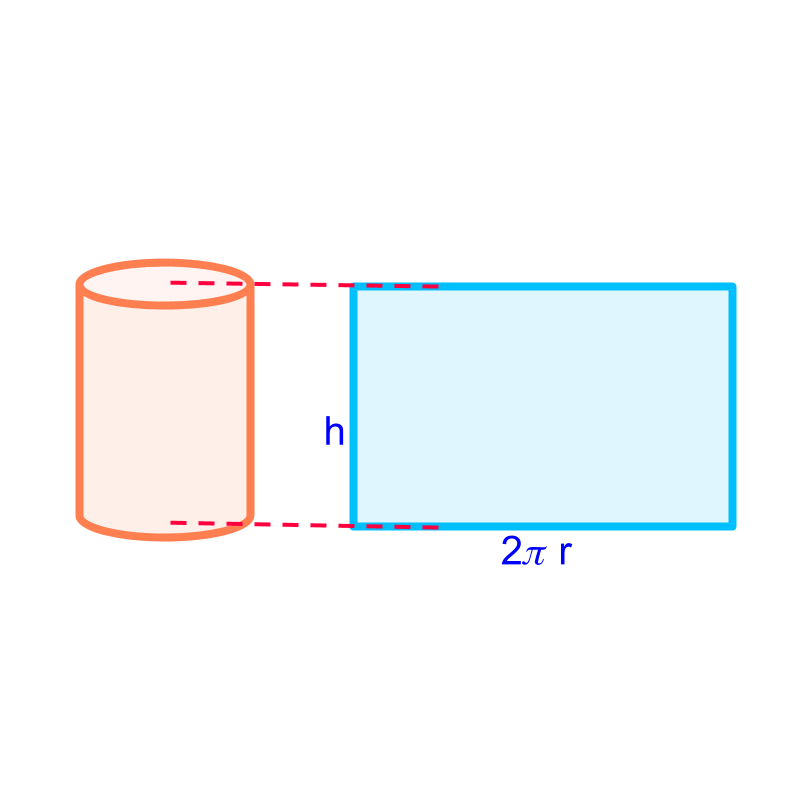Curved Surface Area of Cylinder $=2\pi rh$$= 2 \pi r h$

Surface Area of cylinder $=2\pi r\left(r+h\right)$$= 2 \pi r \left(r + h\right)$

surface-area

Area is the surface-span within a closed 2D-shape. Surface-area is the area of 2D-surfaces enclosing a 3D-shape.

cube

A "cube" is "a 3D shape with $6$$6$ square faces"The surface area of a cube of side $a$$a$ is "$6×{a}^{2}$$6 \times {a}^{2}$". The surface area equals the area of $6$$6$ square faces each having area ${a}^{2}$${a}^{2}$.

cuboidA "cuboid" is "a 3D shape with $6$$6$ rectangular faces"

The surface area of a cuboid of length $l$$l$, breadth $b$$b$, and height $h$$h$ is "$2×\left(lb+bh+hl\right)$$2 \times \left(l b + b h + h l\right)$". The surface area equals the area of $6$$6$ rectangular faces for which area is $lb+lb+bh+bh+hl+hl$$l b + l b + b h + b h + h l + h l$

cylinder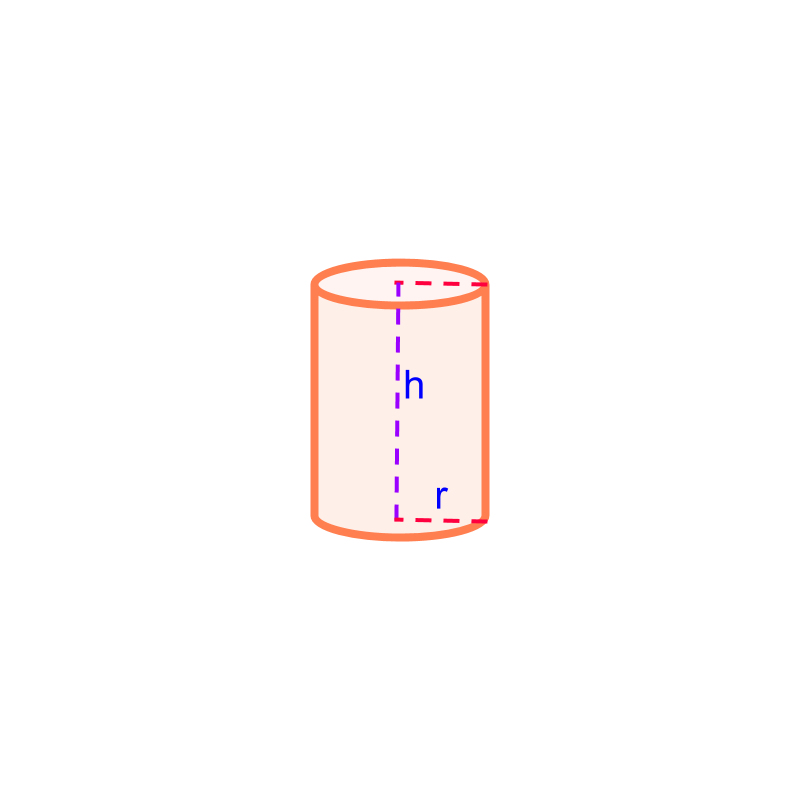Cylinder is a 3D shape that has circular cross-section uniformly along its axis.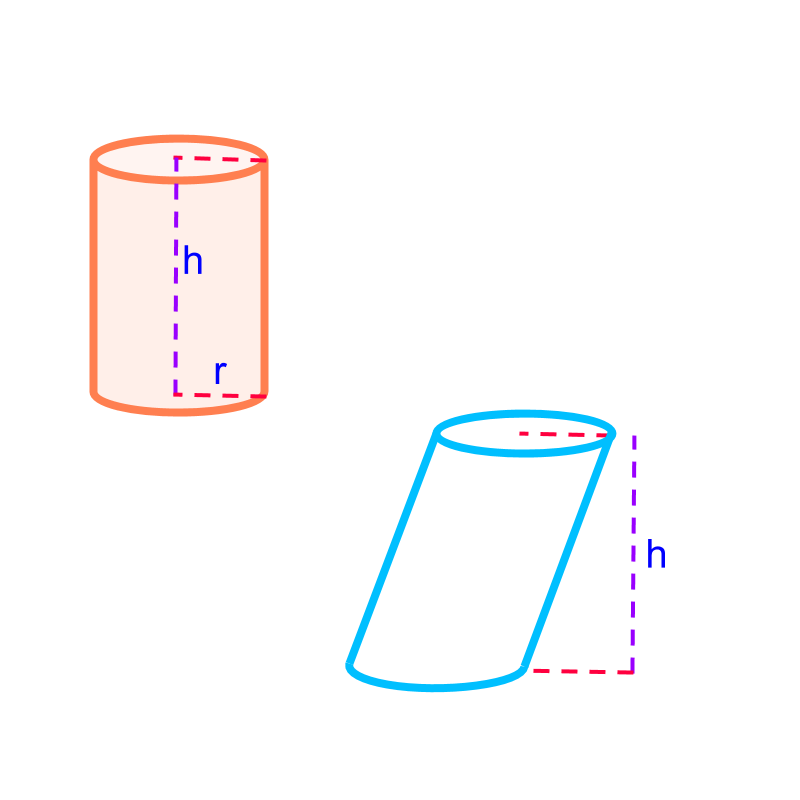When not mentioned, a cylinder is a right-cylinder with it axis at right-angle to the top and bottom faces. The other type is the oblique cylinder, in which the angle between the axis and the top (or bottom) face is not a right-angle. The right cylinder is shown in orange, and oblique cylinder is shown in blue.The surface area of a cylinder of height $h$$h$ and radius $r$$r$ is sum of the areas of ($2$$2$ circles on top and bottom) and the area of the curved surface, which is given as $2×\pi ×{r}^{2}+2×\pi ×r×h$$2 \times \pi \times {r}^{2} + 2 \times \pi \times r \times h$

Note: The cylinder consists of top and bottom circular-faces and a curved surface.The curved surface is visualized into a rectangle of length $2\pi r$$2 \pi r$ and height $h$$h$.

The curved surface area of the cylinder equals the area of the rectangle.

Total surface area of the cylinder

$=$$=$ area of the circle on top and bottom $+$$+$ area of the curved surface

$=2×\pi ×{r}^{2}+2×\pi ×r×h$$= 2 \times \pi \times {r}^{2} + 2 \times \pi \times r \times h$

$=2\pi r\left(r+h\right)$$= 2 \pi r \left(r + h\right)$

example

What is the surface area of a cuboid of length $2$$2$ cm, breadth $3$$3$ cm, and height $4$$4$ cm?
The answer is "$52c{m}^{2}$$52 c {m}^{2}$"

summary

Surface Area of Some Shapes:Surface Area of cube $=6{q}^{2}$$= 6 {q}^{2}$Surface Area of cuboid $=2\left(lb+bh+hl\right)$$= 2 \left(l b + b h + h l\right)$Curved Surface Area of Cylinder $=2\pi rh$$= 2 \pi r h$

Surface Area of cylinder $=2\pi r\left(r+h\right)$$= 2 \pi r \left(r + h\right)$

Outline

The outline of material to learn "Mensuration basics : Length, Area, & Volume" is as follows.

•  Measuring Basics

→   Introduction to Standards

→   Measuring Length

→   Accurate & Approximate Meaures

→   Measuring Area

→   Measuring Volume

→   Conversion between Units of Measure

•  2D shapes

→   Perimeter of Polygons

→   Area of Square & rectangle

→   Area of Triangle

→   Area of Polygons

→   Perimeter and area of a Circle

→   Perimeter & Area of Quadrilaterals

•  3D shapes

→   Surface Area of Cube, Cuboid, Cylinder

→   Volume of Cube, Cuboid, Cylinder